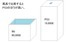Unresolved Detail In Plotted Equations

Sometimes the calculator detects that an equation is too complicated to plot perfectly in a reasonable amount of time. When this happens, the equation is plotted at lower resolution.

235 out of 261 found this helpful
Have more questions? Submit a request

•GD TheProGamer711

Hmmmmmmmmmmmmmm...

\sqrt{bananana}=xy^{3^2}\left(\cos \left(xyx\right)\right)

b=0.299

a=2.571

n=4.954

hit dem play buttons and wtf is happening

•Muellwm

it cant handle this but i want to see what it would look like. is there any way that i can allow it to just take like an hour so i can atleast see it.

y^2-\left(\frac{\sqrt{\sin \left(-200x\right)}}{\sin \left(\left(-200x^{-1}\right)\right)}\right)^2=x^2-\left(\frac{\sqrt{\sin \left(-200y\right)}}{\sin \left(\left(-200y^{-1}\right)\right)}\right)^2

•Andre Cura

\frac{1}{2}<\operatorname{floor}\left(\operatorname{mod}\left(\operatorname{floor}\left(\frac{y}{17}\right)\cdot 2^{-17\cdot \operatorname{mod}\left(\operatorname{floor}\left(y\right),17\right)},2\right)\right)

Tupper's self referential formula. I cannot scale it well

•伊藤那由多

@Muellwm
You can zoom it out or get away from (0,0) to see the good structure.
If you zoom into [-0.1,0.1] scale, you will see square "chunks" with the side length of π/200. Why do you see? Because you inserted -200x and -200y into the square root. Since the numbers in the square roots must be positive, the graph must be in these "chunks." Each chunk structure becomes more complicated as you go closer to zero, because you inserted x^-1 in the equation.
(Just for fun, zoom in to some of the chunks within 0.5<=x<=1 and 0.5<=y<=1)

@Andle Cura
That is just actually a strange binary counter only for y axis and not describes itself.

•t4canty

y=\frac{x^{\cot\left(\sum_{n=1}^{10}\sin\left(x\right)\right)}}{y^2} is pretty nice

•obiwan847

y^2=sin xy

•Oon Han

Here is one:

\frac{\sin x}{\cos x}=\tan x

•Brendon FoxDragon

sin(xx)=cos(y) is pretty cool.

•Cldonkin

i took what Brendon FoxDragon posted and kept going then felt that the center was empty

•Caleb Giger

e^y=\sin\left(\cos\left(\tan x\right)\right) is great

•Caleb Giger

\frac{\sin\left(\cos\left(\tan\left(\csc\left(\sec\left(\cot\left(y\right)\right)\right)\right)\right)\right)}{\sqrt{y^y}}=e^{\cot\left(\sec\left(\csc\left(\tan\left(\cos\left(\sin\left(\ln\left(x\right)\right)\right)\right)\right)\right)\right)} Yep

•Bryan Bielawa

This one was fun

y!=3x^2+8x!+5

•Bryan Bielawa

This one looks like a Christmas tree

y^2=\left|x!\right|

•GD TheProGamer711

\log\left(\operatorname{ceil}\left(x^{\operatorname{floor}\left(y\right)}\right)\right)\ge x

well okay then

•Owen J

This may crash your computer.

•Owen J

These might crash your computer.

https://www.desmos.com/calculator/ujrqjwmhmn

The last one lode is still loding.

•Owen J
•Dillon Strange

x^{-2}y^{20}=10

•Henry Lee

@owen J. The last one is just ^cos(1)

as xyyx/yxxy equals 1, and 1 to any power equals 1.

•Henry Lee
•Apoorv Singh

y=x^tan(xy)

•Owen J

So try these:

Press play on the last one.

•GD TheProGamer711

\sin\left(x^x\right)\ge\sin\left(y^y\right)
Insta chaos in the first quadrant

•Owen J

This is weird:

xxyxxyyyx^y=xyyyxxyxx^x

•Dillon Strange

\frac{\left(\frac{\left(\frac{x!}{x^2}\right)}{\left(\frac{\cos x}{\sin x}\right)}\right)}{\frac{\left(\frac{\sqrt{x}}{x}\right)}{\left(\frac{\tan x}{x^{-1}}\right)}}=\frac{\left(\frac{\left(\frac{y!}{y^2}\right)}{\left(\frac{\cos y}{\sin y}\right)}\right)}{\frac{\left(\frac{\sqrt{y}}{y}\right)}{\left(\frac{\tan y}{y^{-1}}\right)}} is cool if the whole thing could load

Edited by Dillon Strange
•Tristanle216
•Grhumphrey20

Another good one is y=sqrt(sin(x!))

•Thecubicalguy1

It gets REAL deep if you do 1/1/x=x. Creepy vertical lines and dotted ones too. It works with Y too.

•Zak Malamane

t=ln(x) and x=e^t

•247556

x=x^{y^2}

Article is closed for comments.